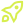Subscribe to our 0800-DEVOPS Newsletter## Not sure where to start? Let our experts guide you. Send us your query through this contact form.## Get in touch## Use the form below to apply for course## Get in touch# Data Analysis

DURATION 2 Days
Learn how to clean, analyse, and visualize data to extract insights and make data-driven decisions.

Overview

This two-day in-person data analytics course is designed to provide business and data analysts with a comprehensive understanding of the practical and theoretical aspects of data analysis. The course will focus on exploratory data analysis using Python, SQL, and MS Excel. Participants will learn how to clean, analyse, and visualize data to extract insights and make data-driven decisions.

Target Audience

• Data Analysts
• Data Scientists
• Data Engineers

Prerequisites

• Basic programming knowledge (preferably Python)
• Basic understanding of databases and SQL
• Familiarity with MS Excel

Content

Introduction to Data Analytics

• The importance of data analytics in business
• Types of data: structured, semi-structured, and unstructured
• Overview of Python, SQL, and MS Excel as data analytics tools

Data Collection

• APIs, web scraping, and databases
• Importing data into Python, SQL, and Excel
• Hands-on exercise:Quick introduction to tools/notebooks

Data Cleaning and Pre-processing

• Handling missing and erroneous values
• Data transformation and normalization
• Feature selection and engineering
• Hands-on exercise: Data cleaning and pre-processing using Python, SQL, and MS Excel

Introduction to Probability and Statistics for Data Analysis

• Basic probability concepts and distributions
• Types of variables
• Descriptive statistics: measures of central tendency and dispersion
• Outliers
• Hands-on exercise:Descriptive statistics on dataset using Python/SQL

Exploratory Data Analysis

• Visualizing data using Python
• Histograms, bar plots, box plots, and scatter plots
• Heatmaps and correlation analysis
• Hands-on exercise: EDA using Python
• SQL for data analysis: aggregation and summary statistics
• GROUP BY, HAVING, and JOIN clauses
• Window functions and analytical functions
• Excel Pivots and Pivots Charts
• Hands-on exercise: EDA using SQL and Excel

Introduction to Machine Learning and Data Preparation for models

• Supervised learning (regression and classification)
• Unsupervised learning (clustering and anomaly detection)
• Model evaluation and validation
• Data preparation
• Handling outliers and missing values
• Standardization
• Normalization
• Handling of categorical variables (one-hot, ordinal encoding)
• Feature Engineering (e.g. extracting time features)
• Hands-on exercise:Performing dataset preparation, building a simple machine learning model using Python and scikit-learn.

Data Analysis Best Practices

• Reproducible research and version control (Git and GitHub)
• Ensuring data quality and integrity
• Communicating results and insights effectively